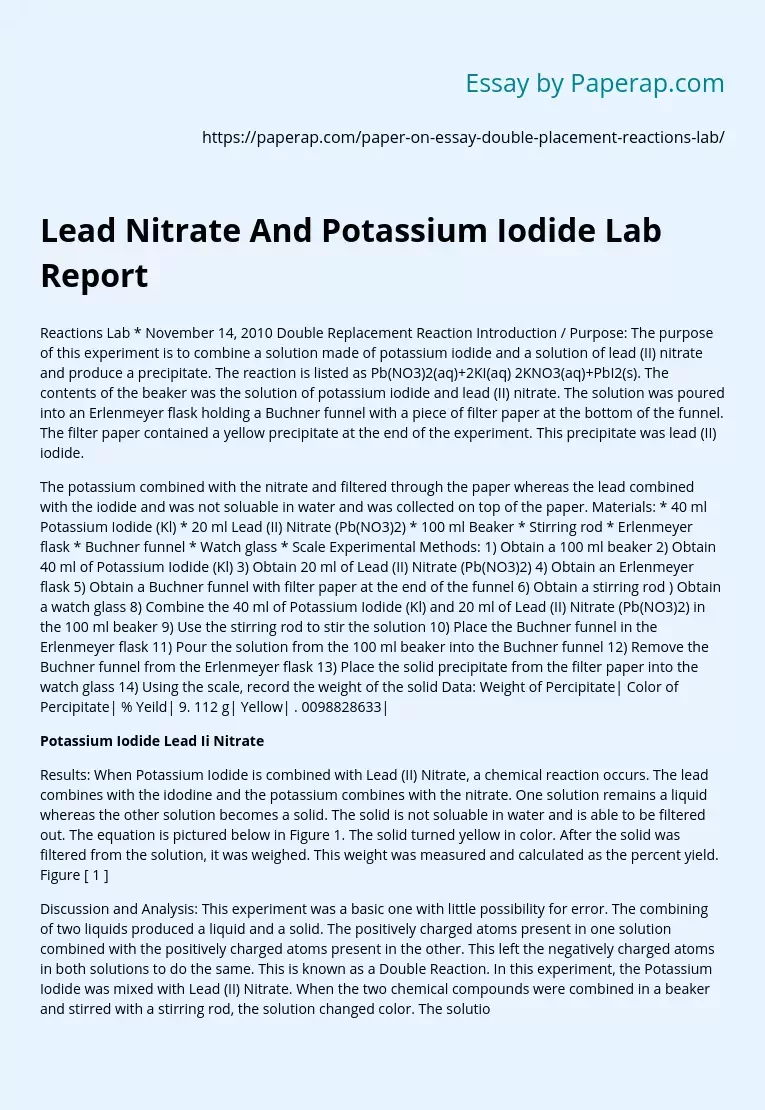# Lead Nitrate And Potassium Iodide Lab Report

Topics: Chemistry

Reactions Lab * November 14, 2010 Double Replacement Reaction Introduction / Purpose: The purpose of this experiment is to combine a solution made of potassium iodide and a solution of lead (II) nitrate and produce a precipitate. The reaction is listed as Pb(NO3)2(aq)+2KI(aq) 2KNO3(aq)+PbI2(s). The contents of the beaker was the solution of potassium iodide and lead (II) nitrate. The solution was poured into an Erlenmeyer flask holding a Buchner funnel with a piece of filter paper at the bottom of the funnel.

The filter paper contained a yellow precipitate at the end of the experiment. This precipitate was lead (II) iodide.

The potassium combined with the nitrate and filtered through the paper whereas the lead combined with the iodide and was not soluable in water and was collected on top of the paper. Materials: * 40 ml Potassium Iodide (Kl) * 20 ml Lead (II) Nitrate (Pb(NO3)2) * 100 ml Beaker * Stirring rod * Erlenmeyer flask * Buchner funnel * Watch glass * Scale Experimental Methods: 1) Obtain a 100 ml beaker 2) Obtain 40 ml of Potassium Iodide (Kl) 3) Obtain 20 ml of Lead (II) Nitrate (Pb(NO3)2) 4) Obtain an Erlenmeyer flask 5) Obtain a Buchner funnel with filter paper at the end of the funnel 6) Obtain a stirring rod ) Obtain a watch glass 8) Combine the 40 ml of Potassium Iodide (Kl) and 20 ml of Lead (II) Nitrate (Pb(NO3)2) in the 100 ml beaker 9) Use the stirring rod to stir the solution 10) Place the Buchner funnel in the Erlenmeyer flask 11) Pour the solution from the 100 ml beaker into the Buchner funnel 12) Remove the Buchner funnel from the Erlenmeyer flask 13) Place the solid precipitate from the filter paper into the watch glass 14) Using the scale, record the weight of the solid Data: Weight of Percipitate| Color of Percipitate| % Yeild| 9.

Get quality help nowWriter LylaVerified

Proficient in: Chemistry5 (876)

“ Have been using her for a while and please believe when I tell you, she never fail. Thanks Writer Lyla you are indeed awesome ”+84 relevant experts are online

112 g| Yellow| . 0098828633|

## Potassium Iodide Lead Ii Nitrate

Results: When Potassium Iodide is combined with Lead (II) Nitrate, a chemical reaction occurs. The lead combines with the idodine and the potassium combines with the nitrate. One solution remains a liquid whereas the other solution becomes a solid. The solid is not soluable in water and is able to be filtered out. The equation is pictured below in Figure 1. The solid turned yellow in color. After the solid was filtered from the solution, it was weighed. This weight was measured and calculated as the percent yield. Figure [ 1 ]

Discussion and Analysis: This experiment was a basic one with little possibility for error. The combining of two liquids produced a liquid and a solid. The positively charged atoms present in one solution combined with the positively charged atoms present in the other. This left the negatively charged atoms in both solutions to do the same. This is known as a Double Reaction. In this experiment, the Potassium Iodide was mixed with Lead (II) Nitrate. When the two chemical compounds were combined in a beaker and stirred with a stirring rod, the solution changed color. The solution in the beaker turned yellow in color.

After stirring the solution, it was poured into a Buchner funnel that had a piece of filter paper at the bottom. This filter paper aided in collecting the precipitate and allowing the remaining liquid solution to pass through. The precipitate that was collected was transferred to a watch glass. After weighing the watch glass, the weight of the solid was calculated. The weight of the solid subtracted from the original weight of the solution gives the weight of the remaining liquid that was filtered through the filter paper. The weight of the solid is used to calculate the percent yield.

The formula used to calculate the percent yield is: = (actual yield/predicted yield) x 100% = (actual yield /((20 ml /1000ml / liter) x GMW of PbI2)) x 100% = (actual yield / (. 2 x 461. 009)) x 100% = (actual yield /9. 22) x 100% = . 0098828633 Conclusions: The experiment went just as planned. The hypothesis was that a precipitate would be yielded as the result of combining two solutions together and passing the solution through filter paper. The solutions combined and reacted just as predicted. The cations and anions combined together and created a precipitate that was yellow in color.

The precipitate was weighed and gave a chance to calculate a percent yield. The data collected was plugged into a formula that was given in the experiment procedures. The weight of the solid precipitate was divided by the predicted yield and then multiplied by 100%. In conclusion, the experiment was a success. Observations Record weight of lead (II) iodide solid (grams): 9. 112 g Calculate % yield = (actual yield / predicted yield) x 100% = (actual yield / ((20 ml /1000 ml/liter) x GMW of PbI2)) x 100 % = (actual yield /(. 02 x 461. 009)) x 100 % = (actual yield / 9. 22) x 100% = 0. 0098828633 g